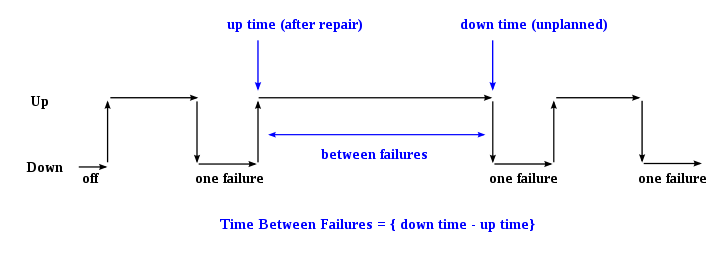# Mean time between failures

﻿
Mean time between failures

Mean time between failures (MTBF) is the predicted elapsed time between inherent failures of a system during operation. MTBF can be calculated as the arithmetic mean (average) time between failures of a system. The MTBF is typically part of a model that assumes the failed system is immediately repaired (MTTR), as a part of a renewal process. This is in contrast to the mean time to failure (MTTF), which measures average time to failures with the modeling assumption that the failed system is not repaired (infinite repair rate).

The definition of MTBF depends on the definition of what is considered a system failure. For complex, repairable systems, failures are considered to be those out of design conditions which place the system out of service and into a state for repair. Failures which occur that can be left or maintained in an unrepaired condition, and do not place the system out of service, are not considered failures under this definition. In addition, units that are taken down for routine scheduled maintenance or inventory control, are not considered within the definition of failure.

## OverviewFor each observation, downtime is the instantaneous time it went down, which is after (i.e. greater than) the moment it went up, uptime. The difference (downtime minus uptime) is the amount of time it was operating between these two events.

MTBF value prediction is an important element in the development of products. Reliability engineers / design engineers, often utilize Reliability Software to calculate products' MTBF according to various methods/standards (MIL-HDBK-217F, Telcordia SR332, Siemens Norm, FIDES,UTE 80-810 (RDF2000), etc.). However, these "prediction" methods are not intended to reflect fielded MTBF as is commonly believed. The intent of these tools is to focus design efforts on the weak links in the design.

## Formal definition of MTBF

By referring to the figure above, the MTBF is the sum of the operational periods divided by the number of observed failures. If the "Down time" (with space) refers to the start of "downtime" (without space) and "up time" (with space) refers to the start of "uptime" (without space), the formula will be:$\text{Mean time between failures} = \text{MTBF} =\frac{\sum{(\text{start of downtime} - \text{start of uptime})}}\text{number of failures}. \!$

The MTBF is often denoted by the Greek letter θ, or$\text{MTBF} = \theta. \!$

The MTBF can be defined in terms of the expected value of the density function ƒ(t)$\text{MTBF} = \int_0^\infty tf(t)\, dt \!$

where ƒ is the density function of time until failure – satisfying the standard requirement of density functions –$\int_0^\infty f(t)\, dt=1. \!$

## Variations of MTBF

There are many variations of MTBF, such as mean time between system aborts (MTBSA) or mean time between critical failures (MTBCF) or mean time between unit replacement (MTBUR). Such nomenclature is used when it is desirable to differentiate among types of failures, such as critical and non-critical failures. For example, in an automobile, the failure of the FM radio does not prevent the primary operation of the vehicle. Mean time to failure (MTTF) is sometimes used instead of MTBF in cases where a system is replaced after a failure, since MTBF denotes time between failures in a system which is repaired.

Wikimedia Foundation. 2010.

### Look at other dictionaries:

• mean time between failures — (MTBF) expected time between malfunctions of an electronic component that is used as an index for determining the reliability of a device …   English contemporary dictionary

• Mean Time Between Failures — MTBF ist die Abkürzung für das englische Mean Time Between Failures, zu deutsch die mittlere Betriebsdauer zwischen Ausfällen. Sie gilt für Einheiten, die instand gesetzt werden; Betriebsdauer meint die Betriebszeit zwischen zwei… …   Deutsch Wikipedia

• Mean Time Between Failures — MTBF; mittlere Betriebsdauer zwischen Ausfällen * * * Mean Time between Failures,   MTBF …   Universal-Lexikon

• mean time between failures — vidutinė trukmė tarp gedimų statusas T sritis automatika atitikmenys: angl. mean time between failures; MTBF vok. mittlerer Abstand zwischen Störungen, m; mittlerer Ausfallabstand, m; mittlerer Störungsabstand, m; MTBF rus. наработка между… …   Automatikos terminų žodynas

• mean time between failures — A statistical measure of the average length of time between the successive failures of equipment or a system. For the specified time interval, the total operating time of the population of material divided by the total number of failures within… …   Aviation dictionary

• mean time between failures —    Abbreviated MTBF. The statistically derived average length of time for which a system component operates before failing. MTBF is expressed in thousands or tens of thousands of hours, also called power on hours, or POH.    See also mean time to …   Dictionary of networking

• mean time between failures —    (MTBF)    The average length of time during which a system or component operates without fault …   IT glossary of terms, acronyms and abbreviations

• mean-time-between failures (MTBF) — Смотри Наработка на отказ …   Энциклопедический словарь по металлургии

• Mean Time Between Overhaul — (Mean) Time Between Overhaul (MTBO bzw. TBO) sind synonyme Bezeichnungen der Englischen Sprache für die durchschnittliche Dauer bis zur Überholung von Geräten, Maschinen oder Motoren. MTBO dient als Maß für die Langlebigkeit von Geräten oder… …   Deutsch Wikipedia

• Mean time between outages — In a system the mean time between outages (MTBO) is the mean time between equipment failures that result in loss of system continuity or unacceptable degradation. The MTBO is calculated by the equation, where MTBF is the nonredundant mean time… …   Wikipedia